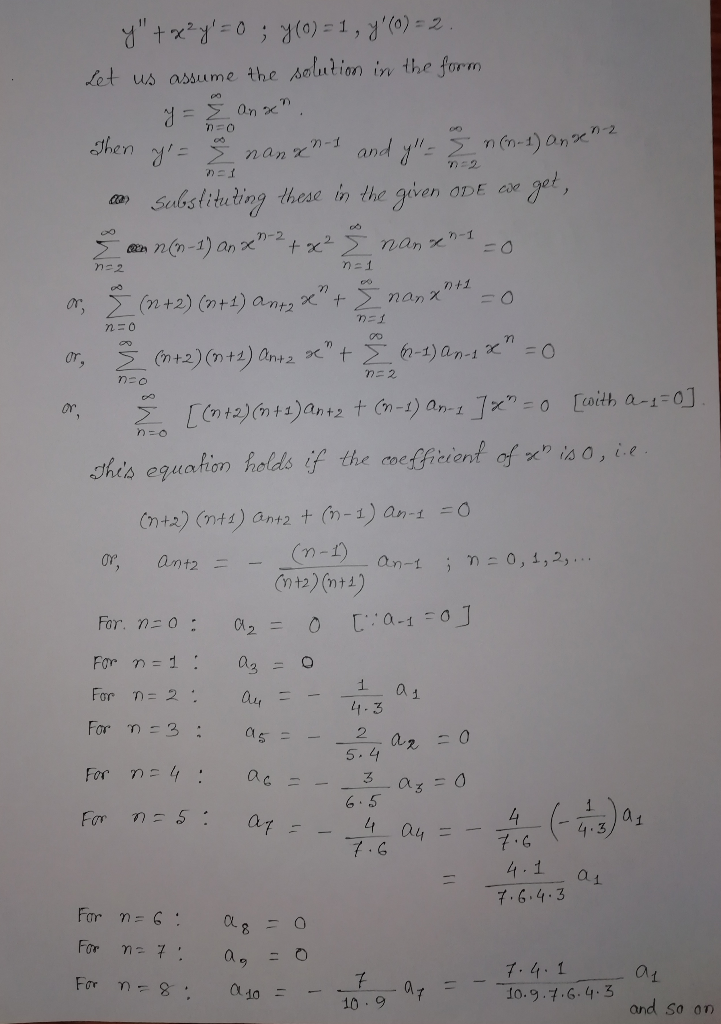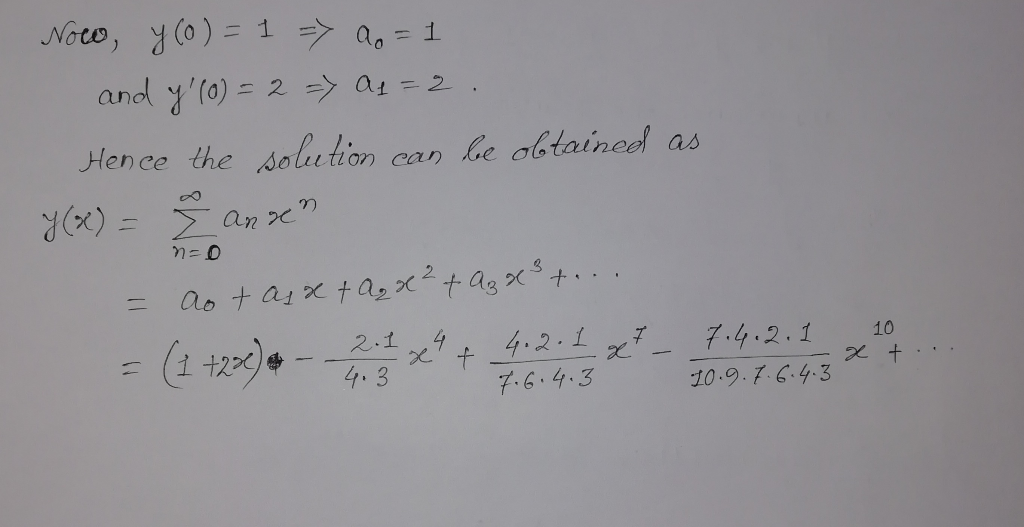# Find a power series solution of this ODE: y''+x^2y'=0 ; y(0)=1 y'(0)= 2

Find a power series solution of this ODE:

y''+x^2y'=0 ; y(0)=1 y'(0)= 2

## Solutions

##### Expert Solution## Related Solutions

##### Find the general power series solution to the following ode: y"-4xy'+(4x2-2)y=0
Find the general power series solution to the following ode: y"-4xy'+(4x2-2)y=0
##### find a power series solution (x-1)y''+y'=0
find a power series solution (x-1)y''+y'=0
##### Find the general power series solution for the differential equation 2x^2y''-xy'+(x^2 +1) y =0 about x=0...
Find the general power series solution for the differential equation 2x^2y''-xy'+(x^2 +1) y =0 about x=0 (Answer should be given to the x^4+r term)
##### Find the general solution to the ODE y'' − y'/x − 4x^2y = x^2 sinh(x^2)
Find the general solution to the ODE y'' − y'/x − 4x^2y = x^2 sinh(x^2)
##### 1)Find the power series solution for the equation y'' − y = x 2)Find the power...
1)Find the power series solution for the equation y'' − y = x 2)Find the power series solution for the equation y'' + (sinx)y = x; y(0) = 0; y'(0) = 1 Provide the recurrence relation for the coefficients and derive at least 3 non-zero terms of the solution.
##### x*y' '+y'-x*y=0 1> use Frobenious series solution to solve the ODE.
x*y' '+y'-x*y=0 1> use Frobenious series solution to solve the ODE.
##### Find the power series solution for the equation y'' + (sinx)y = x; y(0) = 0;...
Find the power series solution for the equation y'' + (sinx)y = x; y(0) = 0; y'(0) = 1 Provide the recurrence relation for the coefficients and derive at least 3 non-zero terms of the solution.
##### . Consider a Bessel ODE of the form: x^2y"+xy'+(x^2-1)y=0 What is the general solution to this...
. Consider a Bessel ODE of the form: x^2y"+xy'+(x^2-1)y=0 What is the general solution to this ODE assuming that the domain of the problem includes x=0? Why?
##### Use power series to solve the initial value problem x^2y''+xy'+x^2y=0, y(0)=1, y'(0)=0
Use power series to solve the initial value problem x^2y''+xy'+x^2y=0, y(0)=1, y'(0)=0
##### Determine step by step power series solution about x=0 for: 4xy'' + 2y' - y =...
Determine step by step power series solution about x=0 for: 4xy'' + 2y' - y = 0. note: final answer must be in terms of coshx and sinhx